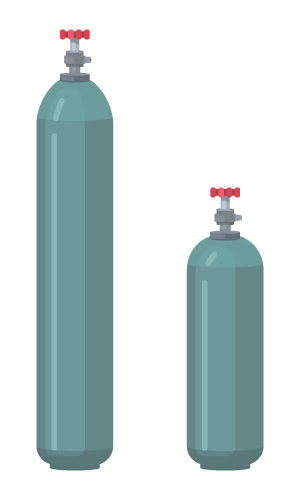# 8.9: Conversions Between Moles and Gas Volume

$$\newcommand{\vecs}{\overset { \rightharpoonup} {\mathbf{#1}} }$$ $$\newcommand{\vecd}{\overset{-\!-\!\rightharpoonup}{\vphantom{a}\smash {#1}}}$$$$\newcommand{\id}{\mathrm{id}}$$ $$\newcommand{\Span}{\mathrm{span}}$$ $$\newcommand{\kernel}{\mathrm{null}\,}$$ $$\newcommand{\range}{\mathrm{range}\,}$$ $$\newcommand{\RealPart}{\mathrm{Re}}$$ $$\newcommand{\ImaginaryPart}{\mathrm{Im}}$$ $$\newcommand{\Argument}{\mathrm{Arg}}$$ $$\newcommand{\norm}{\| #1 \|}$$ $$\newcommand{\inner}{\langle #1, #2 \rangle}$$ $$\newcommand{\Span}{\mathrm{span}}$$ $$\newcommand{\id}{\mathrm{id}}$$ $$\newcommand{\Span}{\mathrm{span}}$$ $$\newcommand{\kernel}{\mathrm{null}\,}$$ $$\newcommand{\range}{\mathrm{range}\,}$$ $$\newcommand{\RealPart}{\mathrm{Re}}$$ $$\newcommand{\ImaginaryPart}{\mathrm{Im}}$$ $$\newcommand{\Argument}{\mathrm{Arg}}$$ $$\newcommand{\norm}{\| #1 \|}$$ $$\newcommand{\inner}{\langle #1, #2 \rangle}$$ $$\newcommand{\Span}{\mathrm{span}}$$$$\newcommand{\AA}{\unicode[.8,0]{x212B}}$$Figure $$\PageIndex{1}$$ (Credit: CK-12 Foundation; Source: CK-12 Foundation; License: CK-12 Curriculum Materials license)

### How can you tell how much gas is in these containers?

Small gas tanks are often used to supply gases for chemistry reactions. A gas gauge will give some information about how much is in the tank, but quantitative estimates are needed so that the reaction will be able to proceed to completion. Knowing how to calculate the necessary parameters for gases is very helpful to avoid running out earlier than desired.

## Conversions Between Moles and Gas Volume

Molar volume at STP can be used to convert from moles to gas volume and from gas volume to moles. The equality of $$1 \: \text{mol} = 22.4 \: \text{L}$$ is the basis for the conversion factor.

##### Example $$\PageIndex{1}$$: Converting Gas Volume to Moles

Many metals react with acids to produce hydrogen gas. A certain reaction produces $$86.5 \: \text{L}$$ of hydrogen gas at STP. How many moles of hydrogen were produced?

###### Known
• $$86.5 \: \text{L} \: \ce{H_2}$$
• $$1 \: \text{mol} = 22.4 \: \text{L}$$
###### Unknown
• moles of H2

Apply a conversion factor to convert from liters to moles.

###### Step 2: Calculate.

$86.5 \: \text{L} \: \ce{H_2} \times \frac{1 \: \text{mol} \: \ce{H_2}}{22.4 \: \text{L} \: \ce{H_2}} = 3.86 \: \text{mol} \: \ce{H_2}\nonumber$

The volume of gas produced is nearly four times larger than the molar volume. The fact that the gas is hydrogen plays no role in the calculation.

##### Example $$\PageIndex{2}$$: Convertig Moles to Gas Volume

What volume does $$4.96 \: \text{mol}$$ of $$\ce{O_2}$$ occupy at STP?

###### Solution

Step 1: List the known quantities and plan the problem.

###### Known
• $$4.96 \: \text{mol} \: \ce{O_2}$$
• $$1 \: \text{mol} = 22.4 \: \text{L}$$
• volume of O2
###### Step 2: Calculate.

$4.96 \: \text{mol} \times 22.4 \: \text{L/mol} = 111.1 \: \text{L}\nonumber$

The volume seems correct given the number of moles.

##### Example $$\PageIndex{3}$$: Converting Volume to Mass

If we know the volume of a gas sample at STP, we can determine how much mass is present. Assume we have $$867 \: \text{L}$$ of $$\ce{N_2}$$ at STP. What is the mass of the nitrogen gas?

###### Known
• $$867 \: \text{L} \: \ce{N_2}$$
• $$1 \: \text{mol} = 22.4 \: \text{L}$$
• Molar mass $$\ce{N_2} = 28.02 \: \text{g/mol}$$
• mass of N2
###### Step 2: Calculate.

We start by determining the number of moles of gas present. We know that 22.4 liters of a gas at STP equals one mole, so:

$867 \: \text{L} \times \frac{1 \: \text{mol}}{22.4 \: \text{L}} = 38.7 \: \text{mol}\nonumber$

We also know the molecular weight of $$\ce{N_2}$$ $$\left( 28.0 \: \text{g/mol} \right)$$, so we can then calculate the weight of nitrogen gas in 867 liters:

$38.7 \: \text{mol} \times \frac{28 \: \text{g}}{1 \: \text{mol}} = 1083.6 \: \text{g} \: \ce{N_2}\nonumber$

In a multi-step problem, be sure that the units check out.

## Summary

• Conversions between moles and volume of a gas are shown.

## Review

1. In the problems above, why was the gas always at standard temperature and pressure?
2. A container contains 45.2 L of N2 gas at STP. How many moles of N2 gas are in the container?
3. If the gas in the previous problem was CH4 gas at STP instead of N2 gas, then how many moles of CH4 gas would there be?

8.9: Conversions Between Moles and Gas Volume is shared under a CC BY-NC license and was authored, remixed, and/or curated by LibreTexts.